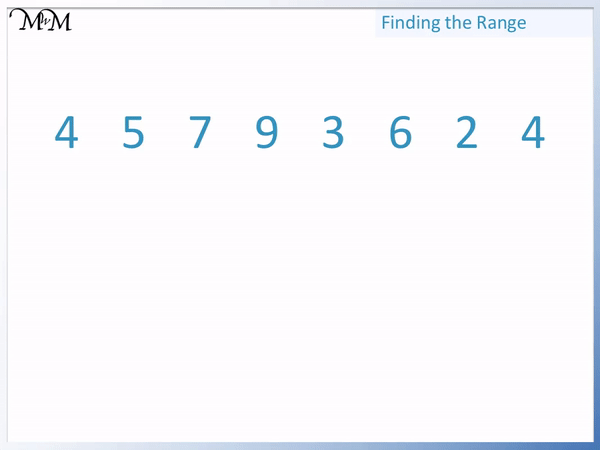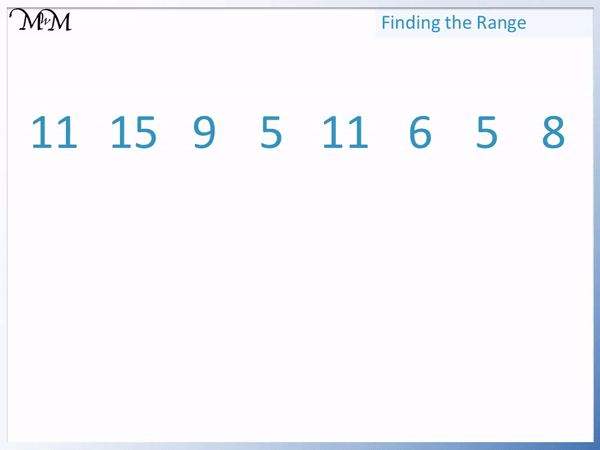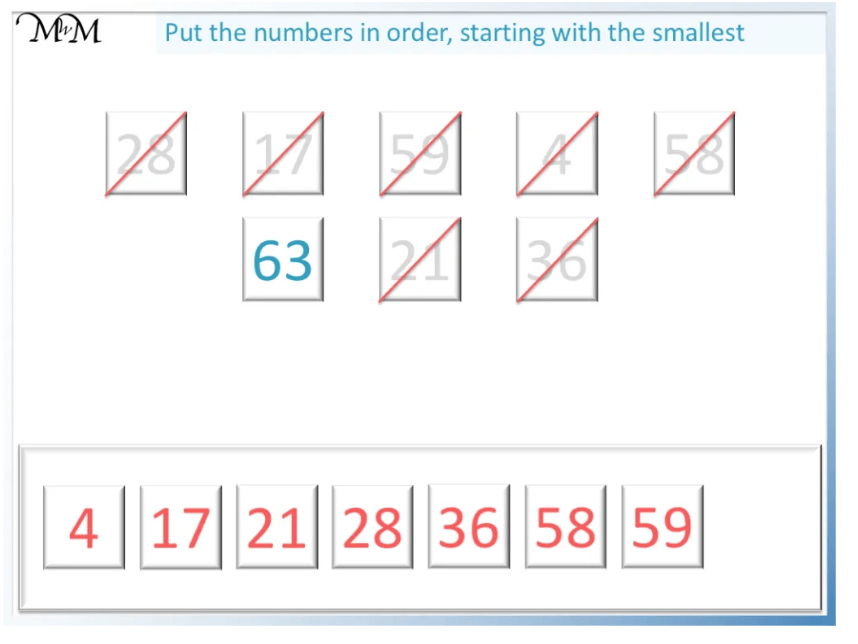# Finding the Range

Finding the Range• The range is the difference between the biggest and smallest numbers in a list.
• To find the range, subtract the smallest number in a list from the largest number.
• The largest number is 9.
• The smallest number is 2.
• 9 – 2 = 7 and so, the range of these numbers is 7.
• The larger the range, the more spread out a set of numbers is.

To find the range of a list of numbers, subtract the smallest number from the largest number.• To find the range, subtract the smallest number from the largest number.
• The largest number is 15.
• The smallest number is 5.
• It does not matter that there are two 5’s in the list, we still use 5 as the smallest value.
• 15 – 5 = 10 and so, the range of these numbers is 10.# Finding the Range

## How to Find the Range of a List of Numbers

To find the range of a list of numbers follow these steps:

1. Find the biggest number in the list.
2. Find the smallest number in the list
3. Subtract the smallest number from the biggest number.
4. This answer is the range.
5. Here are some examples of finding the range of a list of numbers.

In this example we have the list: 4, 5, 7, 9, 3, 6, 2, 4.

The first step is to find the biggest number in the list, which is 9.

The second step is to find the smallest number in the list, which is 2.

The third step is to subtract the smallest number from the biggest number.9 – 2 = 7 and so, the range of these numbers is 7.

Here is a different example of calculating the range.

In this example we have the list: 11, 15, 9, 5, 11, 6, 5, 8.

To find the range, we subtract the smallest number from the largest number.

The largest number is 15 and the smallest number is 5.

It does not matter if there are many of the same number in the list. For example, in this case there are two 5’s. It does not matter because 5 is still the smallest number.15 = 5 = 10 and so, the range of these numbers is 10.

## What is the Range Used For?

The range is a number that is used to indicate how spread out a set of numbers are. The range is the biggest number subtract the smallest number so it tells us how far apart the biggest number and the smallest number are. The range is used to compare different lists of numbers.

The bigger the range, the more spread out the biggest and smallest numbers are.

The smaller the range, the less spread out the biggest and smallest numbers are.

Here are two different lists. We will calculate the range for both examples.

In this list, the largest number is 9 and the smallest number is 2. 9 – 2 = 7 and so the range is 7.In this next list, the largest number is 15 and the smallest number is 5. The range is 15 – 5 = 10.The range can be used to compare the two lists. The range of list 1 was 7 and the range of list 2 was 10.

The numbers in list 1 might be less spread out than the numbers in list 2 because the range of list 1 is smaller than the range of list 2.

The range only takes into account the smallest and largest numbers and so, we cannot describe the spread of the entire list using the range. However it is a very simple way to measure how spread out the numbers in each list are.Now try our lesson Ordering Numbers to 100 where we learn how to put numbers in order from smallest to largest.error: Content is protected !!